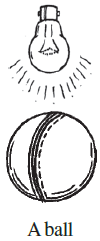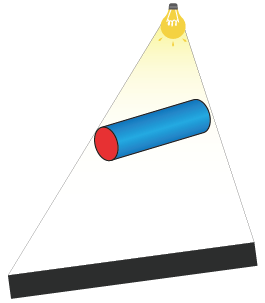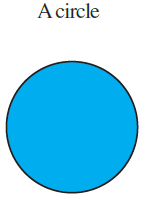## Access Answers to NCERT Class 7 Maths Chapter 15 – Visualising Solid Shapes Exercise 15.4

### Access Answers to NCERT Class 7 Maths Chapter 15 – Visualising Solid Shapes Exercise 15.4

1. A bulb is kept burning just right above the following solids. Name the shape of the shadows obtained in each case. Attempt to give a rough sketch of the shadow. (You may try to experiment first and then answer these questions).

(i)Solution:-The shape of the shadow obtained from a ball is circle.

(ii)Solution:-The shape of the shadow obtained from a cylindrical pipe is rectangle.

(iii)Solution:-The shape of the shadow obtained from a book is rectangle.

2. Here are the shadows of some 3-D objects, when seen under the lamp of an overhead projector. Identify the solid(s) that match each shadow. (There may be multiple answers for these!)

(i)Solution:-

Cricket ball, water bottle cap, Disc

(ii)Solution:-

Die, chalk box etc.

(iii)Solution:-

Birthday cap etc.

(iv)Solution:-

Book, keyboard, Mobile etc.

3. Examine if the following are true statements:

(i) The cube can cast a shadow in the shape of a rectangle.

Solution:-

The above statement is true.

(ii) The cube can cast a shadow in the shape of a hexagon.

Solution:-

The above statement is false.